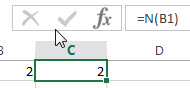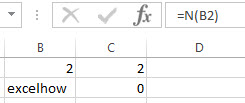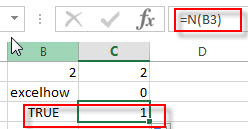# Excel N Function

This post will guide you how to use Excel N function with syntax and examples in Microsoft excel.

### Description

The Excel N function converts a value to a numeric value. And the N function can be used to convert the logical value TRUE as 1 and false value as 0. If you try to convert a text value, and the N function will return 0.

The N function is a build-in function in Microsoft Excel and it is categorized as an Information Function.

The N function is available in Excel 2016, Excel 2013, Excel 2010, Excel 2007, Excel 2003, Excel XP, Excel 2000, Excel 2011 for Mac.

### Syntax

The syntax of the N function is as below:

`=N(value)`

Where the N function argument is:

• Value – This is a required argument. The value that you want to convert.

N converts values listed in the following table.

 If value is or refers to N returns A number That number A date, in one of the built-in date formats available in Microsoft Excel The serial number of that date TRUE 1 FALSE 0 An error value, such as #DIV/0! The error value Anything else 0

### Excel N Function Example

The below examples will show you how to use Excel N Function to convert a value to a number.

#1 to convert the value in Cell B1 that contains a number, and the number will be returned, using the following formula:

`= N (B1)`#2 to convert the value in Cell B2 that contains text, and the zero will be returned, using the following formula:

`= N (B2)`#3 to convert the value in Cell B3 that contains a logical value TRUE, and 1 is returned, using the following formula:

`= N (B3)`### More Excel N Function Examples

• Count Number of Cells with String Length greater than X
You can create a new formula based on the SUMPRODUCT function, the N function and the LEN function to check the string length of each cell and also get the total number that the string length is greater than number 10.……